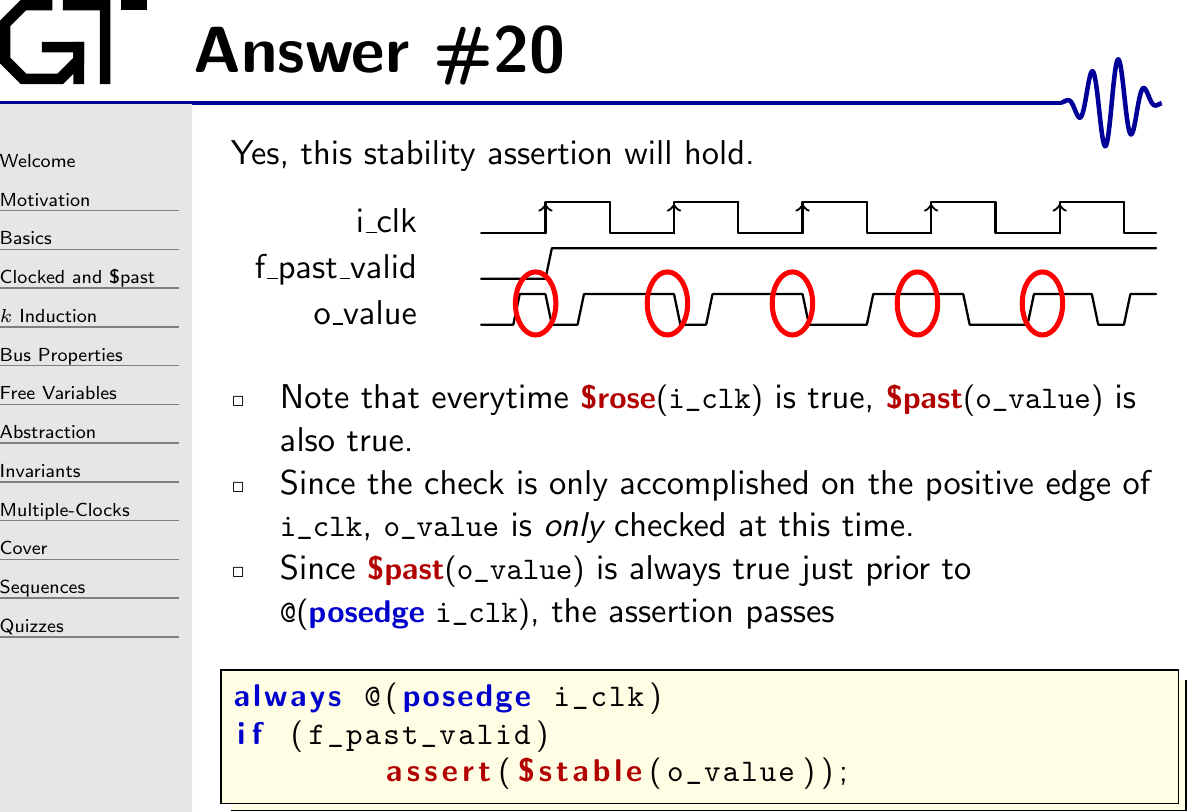The key to understanding what’s going on here lies in understanding how \$stable and \$past work.

\$past, for example, works like a simple registered value.

There’s a couple of things to note in this implementation.

1. `_past_o_value` has no initial value. This is why I use an `f_past_valid` register–to force checks to only take place after the first clock that has given `_past_o_value` a valid value.

2. With any temporal property, such as `\$past`, understanding the clock edge is relevant. In this case, `\$past(o_value)` is defined by the clock edge `posedge i_clk`. If `o_value` is not synchronous with `i_clk`, this edge will probably not make any sense–as you can see from the example above.

Now that we know how `\$past` works, let’s go back and look at the quiz question. If you look carefully, you’ll see that just prior to every clock edge I carefully made certain that `o_value` would be high. That means that every `\$past(o_value)` expression (equivalent to `_past_o_value` above) would be a constant high value–once the first clock edge had passed.

The next key piece to this quiz is that `\$stable(X)` is equivalent to `X == \$past(X)`. Hence, we might rewrite this assertion as:

using our definition of `_past_o_value` above.

The final piece is the `check` register used within the formal engine itself. In this case, that check register would look like:

The formal tool will then use this `assertion_success` register on every time step. If the `assertion_success` register, associated with a given assertion, is ever zero, then the assertion fails.

Herein lies the problem: the `assertion_success` register is only ever set on a positive edge of the clock. Just before that edge, the value is always high. Therefore the assertion passes even though `o_value` changes between clock periods!

If you want to guarantee a value that’s always stable, you might wish instead to use the formal time step for your checks.

That should cover everything except how `f_past_valid` relates. For example, should `f_past_valid` be set on `i_clk` or `formal_timestep_clock`? I’ll leave that last piece, though, as an exercise for the student.